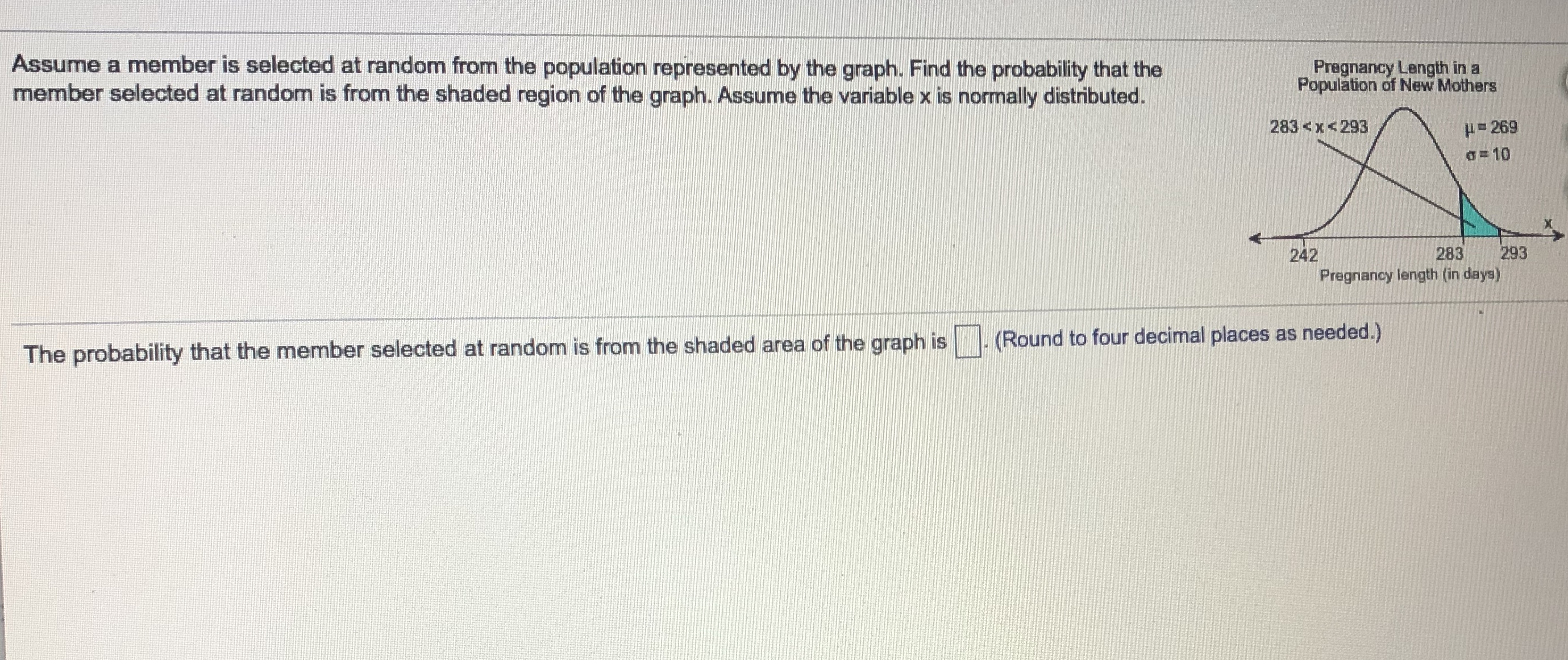# Assume a member is selected at random from the population represented by the graph. Find the probability that themember selected at random is from the shaded region of the graph. Assume the variable x is normally distributed.Pregnancy Length in aPopulation of New Mothers283

Question
21 viewshelp_outlineImage TranscriptioncloseAssume a member is selected at random from the population represented by the graph. Find the probability that the member selected at random is from the shaded region of the graph. Assume the variable x is normally distributed. Pregnancy Length in a Population of New Mothers 283
check_circle

Step 1

It is given that the mean is 269 an...

### Want to see the full answer?

See Solution

#### Want to see this answer and more?

Solutions are written by subject experts who are available 24/7. Questions are typically answered within 1 hour.*

See Solution
*Response times may vary by subject and question.
Tagged in

### Statistics